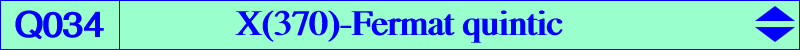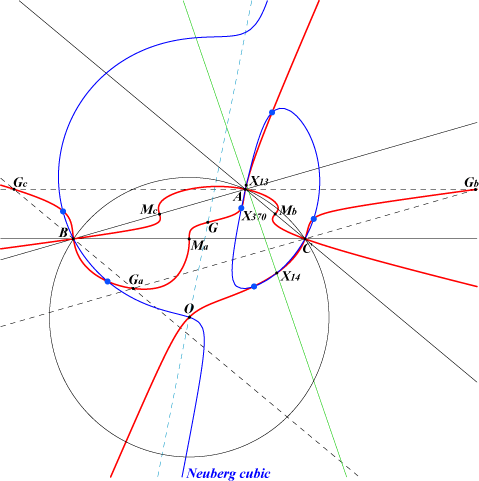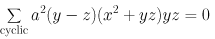X(2), X(3), X(13), X(14), X(370) and its five mates (blue points), see Table 10 complements of these six latter points Ga, Gb, Gc : vertices of the antimedial triangle Ma, Mb, Mc : vertices of the medial triangle CPCC or H-cevian points, see Table 11 foci of the inscribed Steiner ellipse.The "sum" of the three quintics Q036-A, Q036-B, Q036-C is the quintic Q034 which obviously contains X(370) and its five mates. Q034 has three nodes A, B, C where the nodal tangents are the bisectors. It passes through the vertices of the medial and antimedial triangles. The tangents at Ma, Mb, Mc are the perpendicular bisectors of ABC. The tangent at G is the line GK. See the related quartic Q099. See also Table 11 : CPCC points related curves, Table 10 : X(370) and related curves and Table 28 : cevian and anticevian points. Q034 contains the {X}-Cevian points of any X on the Kiepert hyperbola. The anticomplement of Q034 is Q074, the X(370)-Soddy quintic. Locus properties : locus of P such that the Kiepert hyperbola of the cevian triangle of P contains P. locus of P such that the line passing through P and the orthocenter of the cevian triangle of P contains X(3), together with the circumcircle. See the related Q033, Q105.